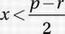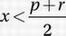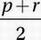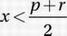# SAT Math Multiple Choice Question 680: Answer and Explanation

### Test Information

Question: 680

5. On a number line, the coordinates of points P and R are p and r, respectively, and p < r. If the point with coordinate x is closer to p than to r, then which of the following statements must be true?

• A.• B.• C. |x - p|< r
• D. |x + p|< r - p

Draw a number line, and to show that p < r, place p to the left of r on the number line. The points that are closer to p than to r are all the points to the left of their midpoint. The midpoint is the average of the endpoints:, so if the point with coordinate x is closer to p than to r, then.